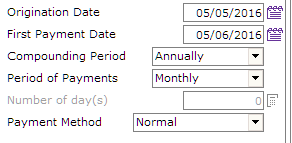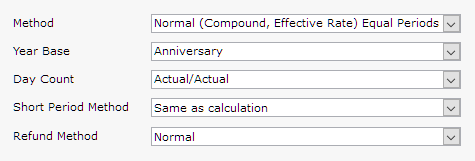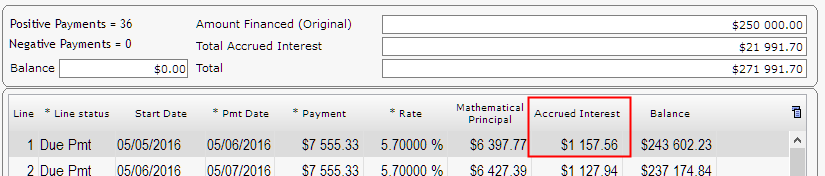# Why am I not getting the right amount of interest for a month?

Question:

Why am I not getting the right amount of interest for a month?

I’ve created a payment schedule for my client for a \$250,000 loan with these dates (my date format is Day/Month/Year, so the first payment is one month after May 5).In my “Advanced” tab I have this:And here is my payment schedule:If I do the calculation by hand I get this:

\$250,000 X 5.7% / 365 days X 30 days = \$1,171.23

Even if there were 31 days I am even further off:

\$250,000 X 5.7% / 365 days X 31 days = \$1,210.27

What’s going on? Am I using the right formula?

This is a common error. People like being able to replicate calculations and they take shortcuts. Doing it the way indicated above was a shortcut BUT the calculation was actually done with what is called Compound interest, Effective rate method, NOT with simple interest as you did the calculation. Compounding was annual (could have been monthly – which is more common when payments are monthly).

The interest calculation formula for compound interest is:

Total compounded interest = P (1 + r/n) (nt) – P

A = future value of the loan, including interest
P = principal amount
r = annual interest rate
n = number of times that interest is compounded per year
t = number of years the money is borrowed for

Our numbers:

P = 250000. r = 5.7/100 = 0.05 (decimal). n = 1. t = 1/12

n=1 since compounded Annually
t= 1/12 since 1 one month (= 0.083333)

If we now plug it in the formula:

A = 250000 (1 + 0.057 / 1) ^ (1(.083333) – 250000 =  \$1157.56.

On the penny!

With Margill you can do simple interest too and even simple interest that is capitalized at various frequencies….